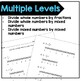Dividing Fractions using Number Line ModelsSubject
Resource Type
Common Core State Standards
Product Rating
File Type

PDF (Acrobat) Document File

Be sure that you have an application to open this file type before downloading and/or purchasing.

4 MB|6+ pages
Share
Also included in:
1. Most students struggle with understanding the concept for dividing fractions. These 2 resources help students visually see the concept of dividing fractions. Included: 4 worksheets increasing in difficulty using a rectangular model for dividing fractions (whole numbers by unit fraction, whole num
\$4.00
\$3.00
Save \$1.00
2. A lot of students struggle with understanding the concept for dividing fractions. These 3 resources help students visually see the concept of dividing fractions.Included:4 worksheets increasing in difficulty using a rectangular model for dividing fractions (whole numbers by unit fraction, whole numb
\$6.00
\$4.50
Save \$1.50
Product Description

Use number line models to help students understand the concept of dividing fractions. Included are:

• 6 printables ranging in difficulty for dividing fractions using a number line (whole number by unit fraction, whole number by fraction, whole number by mixed number, mixed number by mixed number)
• Answer key for all pages
• Teacher directions

Also, be sure to check out my other items for dividing fractions: dividing fractions using models and dividing fractions using models and manipulatives. Or get two of these items in a bundle.

Total Pages
6+ pages
Included
Teaching Duration
N/A
Report this Resource to TpT
Reported resources will be reviewed by our team. Report this resource to let us know if this resource violates TpT’s content guidelines.